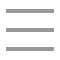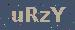个人随笔

Promise 简单入门
2023-02-20 21:46:32

Promise可以封装异步请求，可以让类似文件读取，ajax变得更加优雅，那Promise具体怎么使用呢，下面是我用半天从b站的视频教程中学的基本使用方法，记录一下，后面自己要用也方便

### 一、promise相关知识点

#### 1、Promise的状态

• pending 未决定的
• resolved / fullfilled 成功
• rejected 失败

promise的状态改变

• 调用resolve函数触发pending变为resolved
• 调用reject函数触发或者抛出错误也会触发pending变为rejected

• resolve
• reject

#### 4、链式调用

promise的then()返回一个新的promise，可以用then()进行链式调用，通过then()的链式调用串连多个同步/异步任务

``return new Promise(() = >{});``

### 二、基本使用例子定时任务

#### 1、body里面定义好点击事件

``    <div id="a">setTimeout</div>``

#### 2、js脚本中使用

``    const a = document.querySelector("#a");``    a.addEventListener('click',function(){``    const p = new Promise((resolve,reject)=>{``        setTimeout(()=>{``            let n =Math.random()*100+1;``            if(n<=30){``                //成功``                resolve(n);``            }else{``                //失败``                reject(n);``            }``        },1000);``    });``    p.then((value)=>{``        alert("成功"+value);``    },(reason)=>{``        alert("失败"+reason);``    });``  });``

### 三、基本使用例子ajax

#### 1、body里面定义好点击事件

``<div id="b">ajax</div>``

#### 2、js中脚本

``    const b = document.querySelector("#b");``    b.addEventListener('click',function(){``        const p = new Promise((resolve,reject)=>{``            //1、创建对象``            const xhr = new XMLHttpRequest();``            //2、初始化``            xhr.open('POST','https://www.baidu.com');``            //3、发送``            xhr.send();``            //4、处理响应结果``            xhr.onreadystatechange=function(){``                if(xhr.readState===4){``                    //判断响应状态码为2xx``                    if(xhr.status >=200 && xhr.status<300){``                        //alert("请求成功");``                        resolve(xhr.response);``                    }else{``                        //alert("请求失败"+xhr.status);``                        reject(xhr.status);``                    }``                }``            }``        });``        p.then((value)=>{``            alert(value);``        },(reason)=>{``            alert(reason);``        });``  });``

``  //封装ajax请求``  function sendAjax(url){``        return new Promise((resolve,reject)=>{``        //1、创建对象``        const xhr = new XMLHttpRequest();``        //2、初始化``        xhr.open('POST',url);``        //3、发送``        xhr.send();``        //4、处理响应结果``        xhr.onreadystatechange=function(){``            if(xhr.readState===4){``                //判断响应状态码为2xx``                if(xhr.status >=200 && xhr.status<300){``                    //alert("请求成功");``                    resolve(xhr.response);``                }else{``                    //alert("请求失败"+xhr.status);``                    reject(xhr.status);``                }``            }``        }``    });``  }``  //调用``  sendAjax("https://www.baidu.com").then(value=>{``    alert(value);``  },reason=>{``    alert(reason);``  })``

### 四、async和await结合发送ajax请求

#### 1、body里面点击事件

``<div id="c">async await ajax</div>``

#### 2、js里面简单的代码

``  const c = document.querySelector("#c");``  c.addEventListener('click',async function(){``     let res = await sendAjax('https://www.suibibk.com');``     console.log(res);``  });``

108Copyright : 个人随笔   备案号 : 粤ICP备18099399号